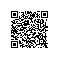# 机器学习算法一览## 2.机器学习算法简述

### 2.1 从机器学习问题角度分类

• 监督学习算法

• 无监督学习

• 半监督学习### 2.2 从算法的功能角度分类

#### 2.2.1 回归算法(Regression Algorithms)• Ordinary Least Squares Regression (OLSR)

• Linear Regression

• Logistic Regression

• Stepwise Regression

• Locally Estimated Scatterplot Smoothing (LOESS)

• Multivariate Adaptive Regression Splines (MARS)

#### 2.2.2 基于实例的算法(Instance-based Algorithms)• k-Nearest Neighbour (kNN)

• Learning Vector Quantization (LVQ)

• Self-Organizing Map (SOM)

• Locally Weighted Learning (LWL)

#### 2.2.3 决策树类算法(Decision Tree Algorithms)• Classification and Regression Tree (CART)

• Iterative Dichotomiser 3 (ID3)

• C4.5 and C5.0 (different versions of a powerful approach)

• Chi-squared Automatic Interaction Detection (CHAID)

• M5

• Conditional Decision Trees

#### 2.2.4 贝叶斯类算法(Bayesian Algorithms)• Naive Bayes

• Gaussian Naive Bayes

• Multinomial Naive Bayes

• Averaged One-Dependence Estimators (AODE)

• Bayesian Belief Network (BBN)

• Bayesian Network (BN)

#### 2.2.5 聚类算法(Clustering Algorithms)• k-Means

• Hierarchical Clustering

• Expectation Maximisation (EM)

#### 2.2.6 关联规则算法(Association Rule Learning Algorithms)• Apriori algorithm

• Eclat algorithm

#### 2.2.7 人工神经网络类算法(Artificial Neural Network Algorithms)• Perceptron

• Back-Propagation

• Radial Basis Function Network (RBFN)

#### 2.2.8 深度学习(Deep Learning Algorithms)• Deep Boltzmann Machine (DBM)

• Deep Belief Networks (DBN)

• Convolutional Neural Network (CNN)

• Stacked Auto-Encoders

#### 2.2.9 降维算法(Dimensionality Reduction Algorithms)• Principal Component Analysis (PCA)

• Principal Component Regression (PCR)

• Partial Least Squares Regression (PLSR)

• Sammon Mapping

• Multidimensional Scaling (MDS)

• Linear Discriminant Analysis (LDA)

• Mixture Discriminant Analysis (MDA)

• Flexible Discriminant Analysis (FDA)

#### 2.2.10 模型融合算法(Ensemble Algorithms)• Random Forest

• Boosting

• Bootstrapped Aggregation (Bagging)

• Stacked Generalization (blending)

• Gradient Boosted Regression Trees (GBRT)

### 2.3 机器学习算法使用图谱

scikit-learn作为一个丰富的python机器学习库，实现了绝大多数机器学习的算法，有相当多的人在使用，于是我这里很无耻地把machine learning cheat sheet for sklearn搬过来了，原文可以看这里。哈哈，既然讲机器学习，我们就用机器学习的语言来解释一下，这是针对实际应用场景的各种条件限制，对scikit-learn里完成的算法构建的一颗决策树，每一组条件都是对应一条路径，能找到相对较为合适的一些解决方法，具体如下：## 3. 机器学习问题解决思路

• 拿到数据后怎么了解数据(可视化)

• 选择最贴切的机器学习算法

• 定位模型状态(过/欠拟合)以及解决方法

• 大量极的数据的特征分析与可视化

• 各种损失函数(loss function)的优缺点及如何选择

### 3.1 数据与可视化### 3.2 机器学习算法选择#### 3.2.1 过拟合的定位与解决

• 增大样本量• 减少特征的量(只用我们觉得有效的特征)(2)神经网络中减少神经网络的层数和每层的结点数 (c)SVM中增加RBF-kernel的bandwidth等方式来降低模型的复杂度。

• 增强正则化作用(比如说这里是减小LinearSVC中的C参数)

正则化是我认为在不损失信息的情况下，最有效的缓解过拟合现象的方法。• l2正则化，它对于最后的特征权重的影响是，尽量打散权重到每个特征维度上，不让权重集中在某些维度上，出现权重特别高的特征。

• 而l1正则化，它对于最后的特征权重的影响是，让特征获得的权重稀疏化，也就是对结果影响不那么大的特征，干脆就拿不着权重。#### 3.2.2 欠拟合定位与解决• 调整你的特征(找更有效的特征！！)
比如说我们观察完现在的数据分布，然后我们先对数据做个映射：• 使用更复杂一点的模型(比如说用非线性的核函数)
我们对模型稍微调整了一下，用了一个复杂一些的非线性rbf kernel：### 3.3 关于大数据样本集和高维特征空间

#### 3.3.1 大数据情形下的模型选择与学习曲线

StandardScaler每次只使用一部分(mini-batch)做训练，在这种情况下，我们使用交叉验证(cross-validation)并不是很合适，我们会使用相对应的progressive validation：简单解释一下，estimator每次只会拿下一个待训练batch在本次做评估，然后训练完之后，再在这个batch上做一次评估，看看是否有优化。#### 3.3.2 大数据量下的可视化PCA降维### 3.4 损失函数的选择• 0-1损失函数(zero-one loss)非常好理解，直接对应分类问题中判断错的个数。但是比较尴尬的是它是一个非凸函数，这意味着其实不是那么实用。

• hinge loss(SVM中使用到的)的健壮性相对较高(对于异常点/噪声不敏感)。但是它没有那么好的概率解释。

• log损失函数(log-loss)的结果能非常好地表征概率分布。因此在很多场景，尤其是多分类场景下，如果我们需要知道结果属于每个类别的置信度，那这个损失函数很适合。缺点是它的健壮性没有那么强，相对hinge loss会对噪声敏感一些。

• 感知损失(perceptron loss)可以看做是hinge loss的一个变种。hinge loss对于判定边界附近的点(正确端)惩罚力度很高。而perceptron loss，只要样本的判定类别结果是正确的，它就是满意的，而不管其离判定边界的距离。优点是比hinge loss简单，缺点是因为不是max-margin boundary，所以得到模型的泛化能力没有hinge loss强。

## 4. 总结使用钉钉扫一扫加入圈子
+ 订阅